Math Olympiad Test: Arithmetic Progression- 1

# Math Olympiad Test: Arithmetic Progression- 1 - Class 10

Test Description

## 10 Questions MCQ Test Olympiad Preparation for Class 10 - Math Olympiad Test: Arithmetic Progression- 1

Math Olympiad Test: Arithmetic Progression- 1 for Class 10 2023 is part of Olympiad Preparation for Class 10 preparation. The Math Olympiad Test: Arithmetic Progression- 1 questions and answers have been prepared according to the Class 10 exam syllabus.The Math Olympiad Test: Arithmetic Progression- 1 MCQs are made for Class 10 2023 Exam. Find important definitions, questions, notes, meanings, examples, exercises, MCQs and online tests for Math Olympiad Test: Arithmetic Progression- 1 below.
 1 Crore+ students have signed up on EduRev. Have you?
Math Olympiad Test: Arithmetic Progression- 1 - Question 1

### If S1, S2, S3, …. Sm are the sum of n terms of m arithmetic progression whose first terms are 1, 2, 3, …. m and common differences are 1, 3, 5, … (2m - 1) respectively. What is the value of S1 + S2 + …. Sm?

Detailed Solution for Math Olympiad Test: Arithmetic Progression- 1 - Question 1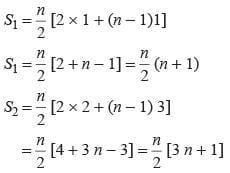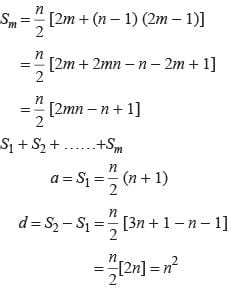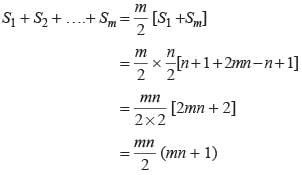Math Olympiad Test: Arithmetic Progression- 1 - Question 2

### If the 3rd and 7th terms of an arithmetic progression are 17 and 27 respectively. Find the first term of arithmetic progression.

Detailed Solution for Math Olympiad Test: Arithmetic Progression- 1 - Question 2

Let a be the first term and d be the common difference
a + 2d = 17
a + 6d = 27
Solving these eqns we get a = 12

Math Olympiad Test: Arithmetic Progression- 1 - Question 3

### If four numbers in arithmetic progression are such that their sum is 50 and the greatest number is 4 times the least. Which number is the least one?

Detailed Solution for Math Olympiad Test: Arithmetic Progression- 1 - Question 3

Let the numbers are a -3d, a - d, a + d, a + 3d
a - 3d + a - d + a + d + a + 3d = 50
⇒ 4a = 50 ⇒ a = 50/4 = 25/2
a + 3d = 4(a - 3d )
⇒ a + 3d = 4a - 12d
⇒ 3a = 15d
⇒ a = 5d
⇒ 25/2 = 5d
⇒ d = 5/2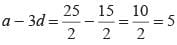Math Olympiad Test: Arithmetic Progression- 1 - Question 4

If 8th term of an arithmetic progression is 31 and its 15th term is 16 more than 11th term of the arithmetic progression. What is the first term of that arithmetic progression?

Detailed Solution for Math Olympiad Test: Arithmetic Progression- 1 - Question 4

Let a be the first term and d be the common difference
a + 7d = 31
a + 14d = a + 10d + 16
a + 14d - a - 10d = 16
⇒ d = 4
∴ a = 31 - 7d = 31 - 28 = 3

Math Olympiad Test: Arithmetic Progression- 1 - Question 5

What is the sum of first 30 terms of the arithmetic progression whose 2nd term is 8 and 4th term is 14?

Detailed Solution for Math Olympiad Test: Arithmetic Progression- 1 - Question 5

Let a be the first term and d be common difference
∴ a + d = 8; a + 3d = 14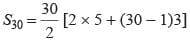= 15 [10 + 87] = 15 × 97 = 1455

Math Olympiad Test: Arithmetic Progression- 1 - Question 6

Find the sum of first 25 terms of an arithmetic progression whose nth term is given by 7 - 3n.

Detailed Solution for Math Olympiad Test: Arithmetic Progression- 1 - Question 6

tn = 7 - 3n
t1 = 7 - 3 × 1 = 4 ⇒ a = 4
t2 = 7 - 3 × 2 = 1
d = 1 - 4 = -3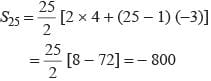Math Olympiad Test: Arithmetic Progression- 1 - Question 7

The first term of an arithmetic progression is 2 and the last term is 50. The sum of all these terms is 442. What is the common difference?

Detailed Solution for Math Olympiad Test: Arithmetic Progression- 1 - Question 7

a = 2 , l = 50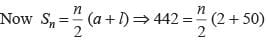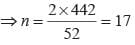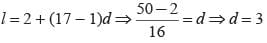Math Olympiad Test: Arithmetic Progression- 1 - Question 8

The sum of the three numbers in arithmetic progression is 12. The sum of their cubes is 288. What are the numbers?

Detailed Solution for Math Olympiad Test: Arithmetic Progression- 1 - Question 8

Let the numbers be a - d, a, a + d.
a - d + a + a + d = 12 ⇒ 3a = 12 ⇒ a = 4
and (a - d)3 + a3 + (a + d)3 = 288
a3 - d3 - 3ad(a - d) + a3 + a3 + d3 + 3ad(a + d) = 288
a3 - d3 - 3ad(a - d) + a3 + a3 + a3 + 3ad + 3ad2 = 288
⇒ 3a3 + 6ad2 = 288 ⇒ 3 × 43 + 6 × 4 × d2 = 288
⇒ 24d2 = 288 - 192
⇒ d2 = 95/24 = 4
⇒ d = ±2

Math Olympiad Test: Arithmetic Progression- 1 - Question 9

If the sum of n terms of an Arithmetic Progression is given by Sn = 3n2 + 2n. Find its first term and common difference.

Detailed Solution for Math Olympiad Test: Arithmetic Progression- 1 - Question 9

Given Sn = 3n2 + 2n
∴ S1 = 3 × 12 + 2 × 1 = 5
S2 = 3 × 22 + 2 × 2 = 16
The sequence is 5, 11, …..
a = 5 , d = 11 - 5 = 6

Math Olympiad Test: Arithmetic Progression- 1 - Question 10

How many terms are there in the Arithmetic Progression?
7, 11, 15, …..139?

Detailed Solution for Math Olympiad Test: Arithmetic Progression- 1 - Question 10

Given AP is 7, 11, 15, ….139
Here a = 7, d = 11 - 7 = 4, l = 139
l = a + (n - 1) d
⇒ 139 = 7 + (n - 1) 4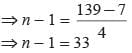⇒ n = 34

## Olympiad Preparation for Class 10

11 videos|36 docs|201 tests